Miscellaneous

Chapter 8 Class 11 Sequences and Series
Serial order wise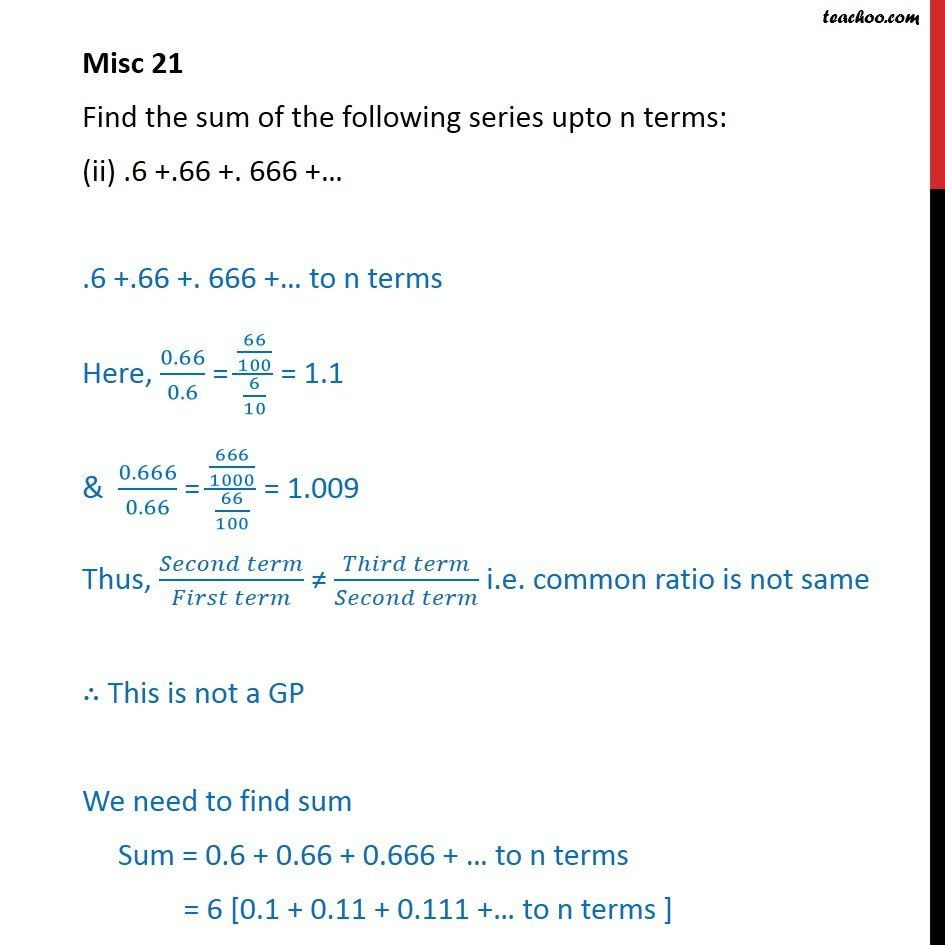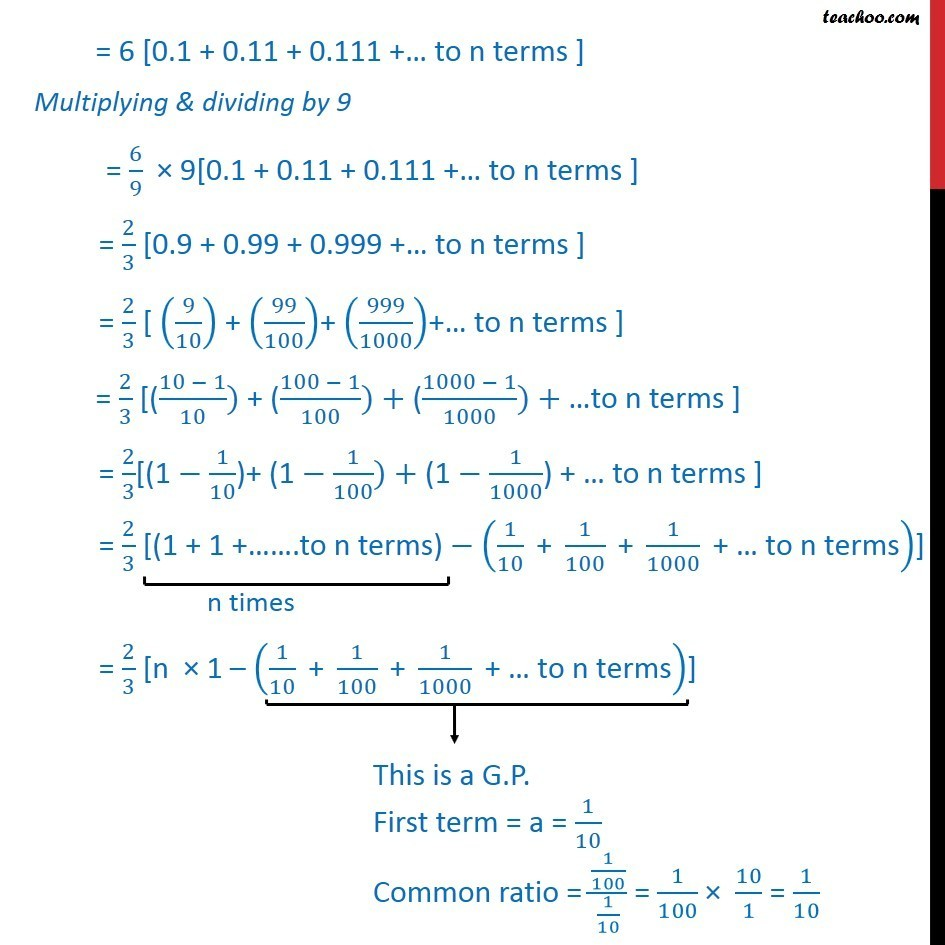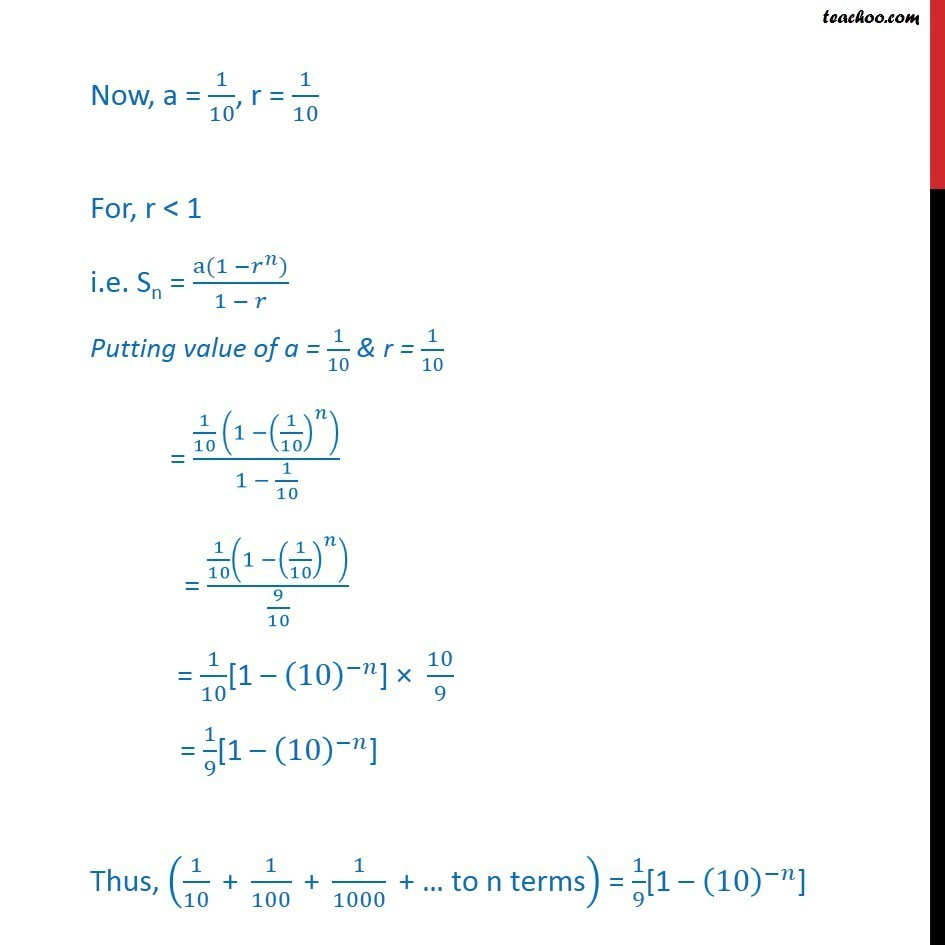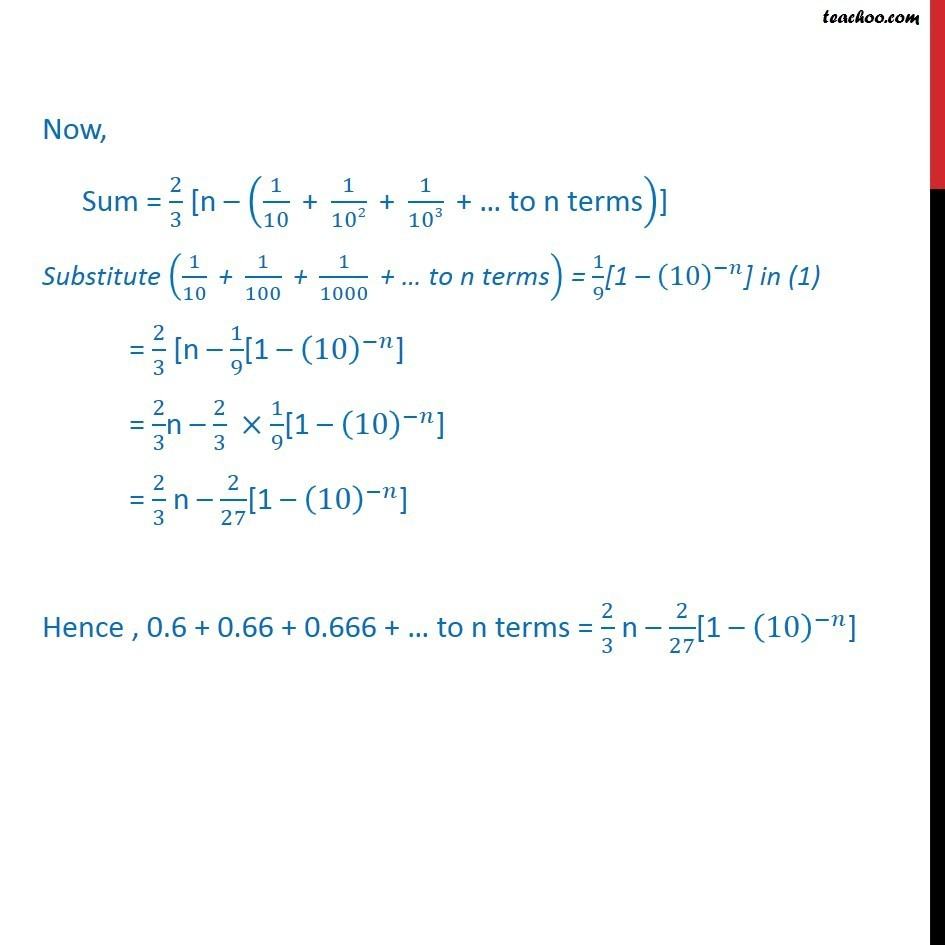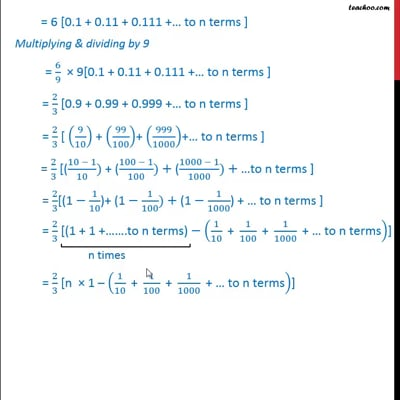This video is only available for Teachoo black users

Learn in your speed, with individual attention - Teachoo Maths 1-on-1 Class

### Transcript

Misc 21 Find the sum of the following series upto n terms: (ii) .6 +.66 +. 666 +… .6 +.66 +. 666 +… to n terms Here, 0.66/0.6 = (66/100)/(6/10) = 1.1 & 0.666/0.66 = (666/1000)/(66/100) = 1.009 Thus, (𝑆𝑒𝑐𝑜𝑛𝑑 𝑡𝑒𝑟𝑚)/(𝐹𝑖𝑟𝑠𝑡 𝑡𝑒𝑟𝑚) ≠ (𝑇ℎ𝑖𝑟𝑑 𝑡𝑒𝑟𝑚)/(𝑆𝑒𝑐𝑜𝑛𝑑 𝑡𝑒𝑟𝑚) i.e. common ratio is not same ∴ This is not a GP We need to find sum Sum = 0.6 + 0.66 + 0.666 + … to n terms = 6 [0.1 + 0.11 + 0.111 +… to n terms ] = 6 [0.1 + 0.11 + 0.111 +… to n terms ] Multiplying & dividing by 9 = 6/9 × 9[0.1 + 0.11 + 0.111 +… to n terms ] = 2/3 [0.9 + 0.99 + 0.999 +… to n terms ] = 2/3 [ (9/10) + (99/100)+ (999/1000)+… to n terms ] = 2/3 [((10 − 1)/10) + ((100 − 1)/100)+ ((1000 − 1)/1000)+ …to n terms ] = 2/3[(1 − 1/10)+ (1 − 1/100)+ (1 − 1/1000) + … to n terms ] = 2/3 [(1 + 1 +…….to n terms) − (1/10 " + " 1/100 " + " 1/1000 " + … to n terms" )] = 2/3 [n × 1 – (1/10 " + " 1/100 " + " 1/1000 " + … to n terms" )] Now, a = 1/10, r = 1/10 For, r < 1 i.e. Sn = (a(1 −𝑟^𝑛))/(1 − 𝑟) Putting value of a = 1/10 & r = 1/10 = (1/10 (1 −(1/10)^𝑛 ))/(1 − 1/10) = (1/10 (1 −(1/10)^𝑛 ))/(9/10) = 1/10[1 – (10)^(−𝑛)] × 10/9 = 1/9[1 – (10)^(−𝑛)] Thus, (1/10 " + " 1/100 " + " 1/1000 " + … to n terms" ) = 1/9[1 – (10)^(−𝑛)] Now, Sum = 2/3 [n – (1/10 " + " 1/102 " + " 1/103 " + … to n terms" )] Substitute (1/10 " + " 1/100 " + " 1/1000 " + … to n terms" ) = 1/9[1 – (10)^(−𝑛)] in (1) = 2/3 [n – 1/9[1 – (10)^(−𝑛)] = 2/3n – 2/3 ×1/9[1 – (10)^(−𝑛)] = 2/3 n – 2/27[1 – (10)^(−𝑛)] Hence , 0.6 + 0.66 + 0.666 + … to n terms = 2/3 n – 2/27[1 – (10)^(−𝑛)]